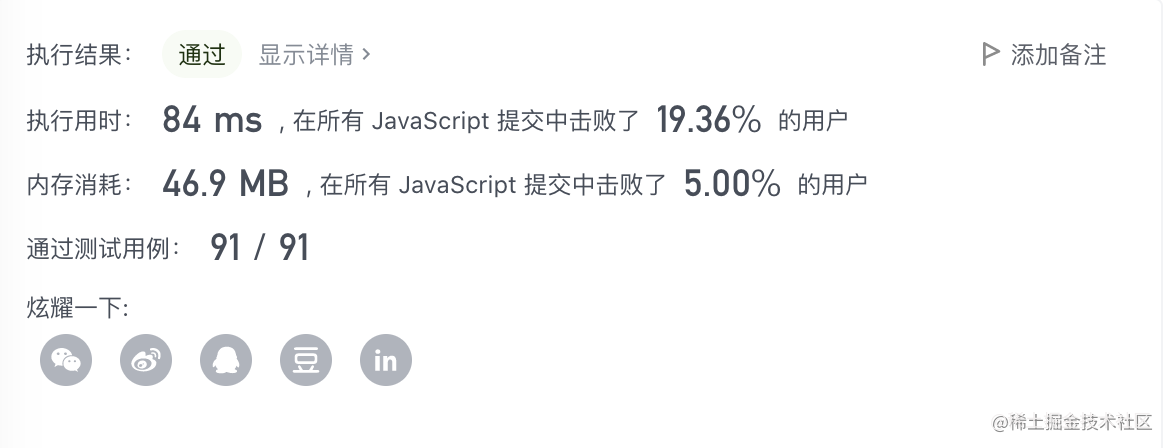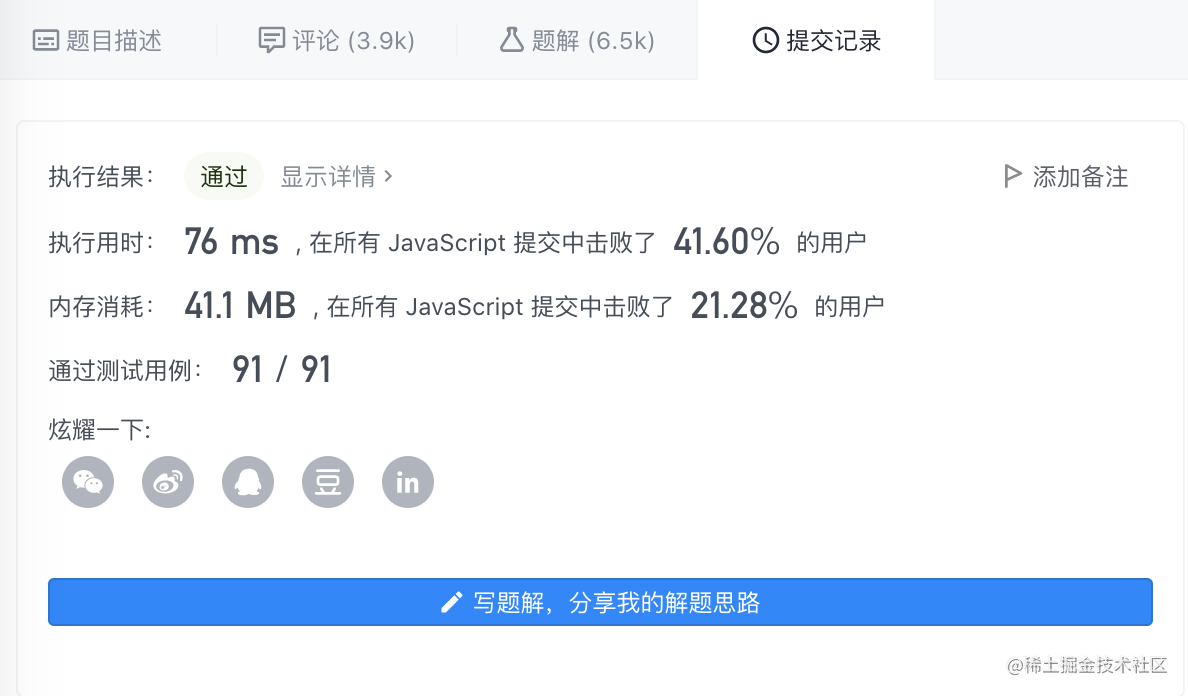# 面试官：你都工作3年了，这个算法题都不会？## 有效的括号问题

1. 左括号必须用相同类型的右括号闭合。
2. 左括号必须以正确的顺序闭合。

``````

### 解题信息

1. 字符串s的长度一定是偶数，不可能是奇数(`一对对匹配`)。
2. `右括号`前面一定跟着`左括号`，才符合匹配条件，具备对称性。
3. `右括号`前面如果不是左括号，一定不是有效的括号。

### 暴力消除法

``````输入：s = "{[()]}"

``````const isValid = (s) => {
while (true) {
let len = s.length
// 将字符串按照匹配对，挨个替换为''
s = s.replace('{}', '').replace('[]', '').replace('()', '')
// 有两种情况s.length会等于len
// 1. s匹配完了，变成了空字符串
// 2. s无法继续匹配，导致其长度和一开始的len一样，比如({],一开始len是3，匹配完还是3，说明不用继续匹配了，结果就是false
if (s.length === len) {
return len === 0
}
}
}### 栈解题法

``````abc
cba

``````输入：s = "{[()]}"

``````const isValid = (s) => {
// 空字符串符合条件
if (!s) {
return true
}

const leftToRight = {
'(': ')',
'[': ']',
'{': '}'
}
const stack = []

for (let i = 0, len = s.length; i < len; i++) {
const ch = s[i]
// 左括号
if (leftToRight[ch]) {
stack.push(ch)
} else {
// 右括号开始匹配
// 1. 如果栈内没有左括号，直接false
// 2. 有数据但是栈顶元素不是当前的右括号
if (!stack.length || leftToRight[ stack.pop() ] !== ch) {
return false
}
}
}

// 最后检查栈内还有没有元素，有说明还有未匹配则不符合
return !stack.length
}## 结尾

••• 子弈
3年前
• 童欧巴
3年前
•• CUGGZ
2年前• 伊人a
1年前•• ConardLi
3年前
• 阿崔cxr
1年前
• ConardLi
3年前
• vortesnail
1年前
• 童欧巴
3年前